# Selina Solutions Concise Mathematics Class 6 Chapter 16: Percent (Percentage) Exercise 16(A)

Selina Solutions Concise Mathematics Class 6 Chapter 16 Percent (Percentage) Exercise 16(A) explains the basic concepts, along with the steps to be followed in expressing the given statement as percent. In a subject like Mathematics, students need a better hold on the concepts covered, as per the latest syllabus. The solutions provide students with important formulas and shortcut tricks in solving exercise wise problems with ease. For better academic performance, students can make use of Selina Solutions Concise Mathematics Class 6 Chapter 16 Percent (Percentage) Exercise 16(A) PDF, from the links available below.

## Selina Solutions Concise Mathematics Class 6 Chapter 16: Percent (Percentage) Exercise 16(A) Download PDF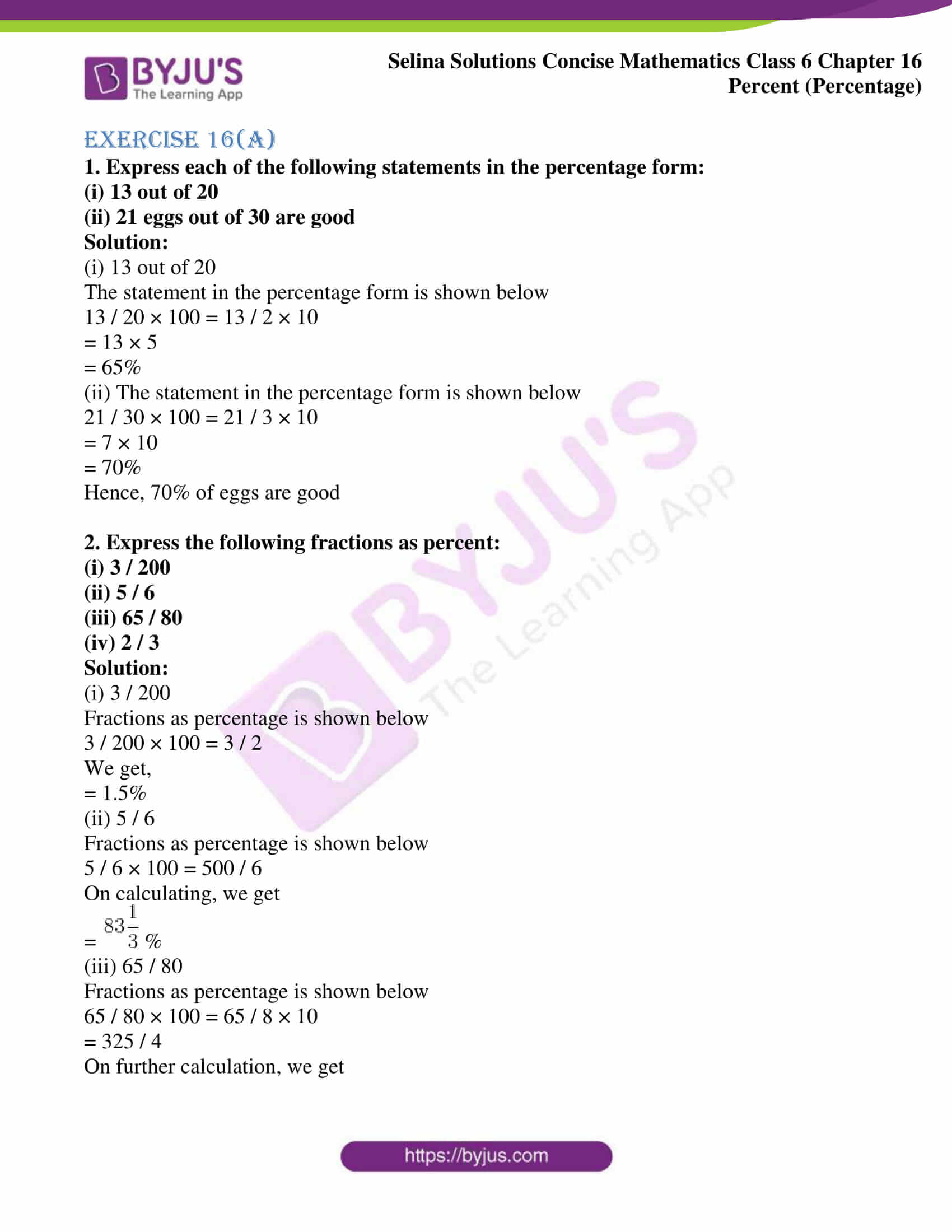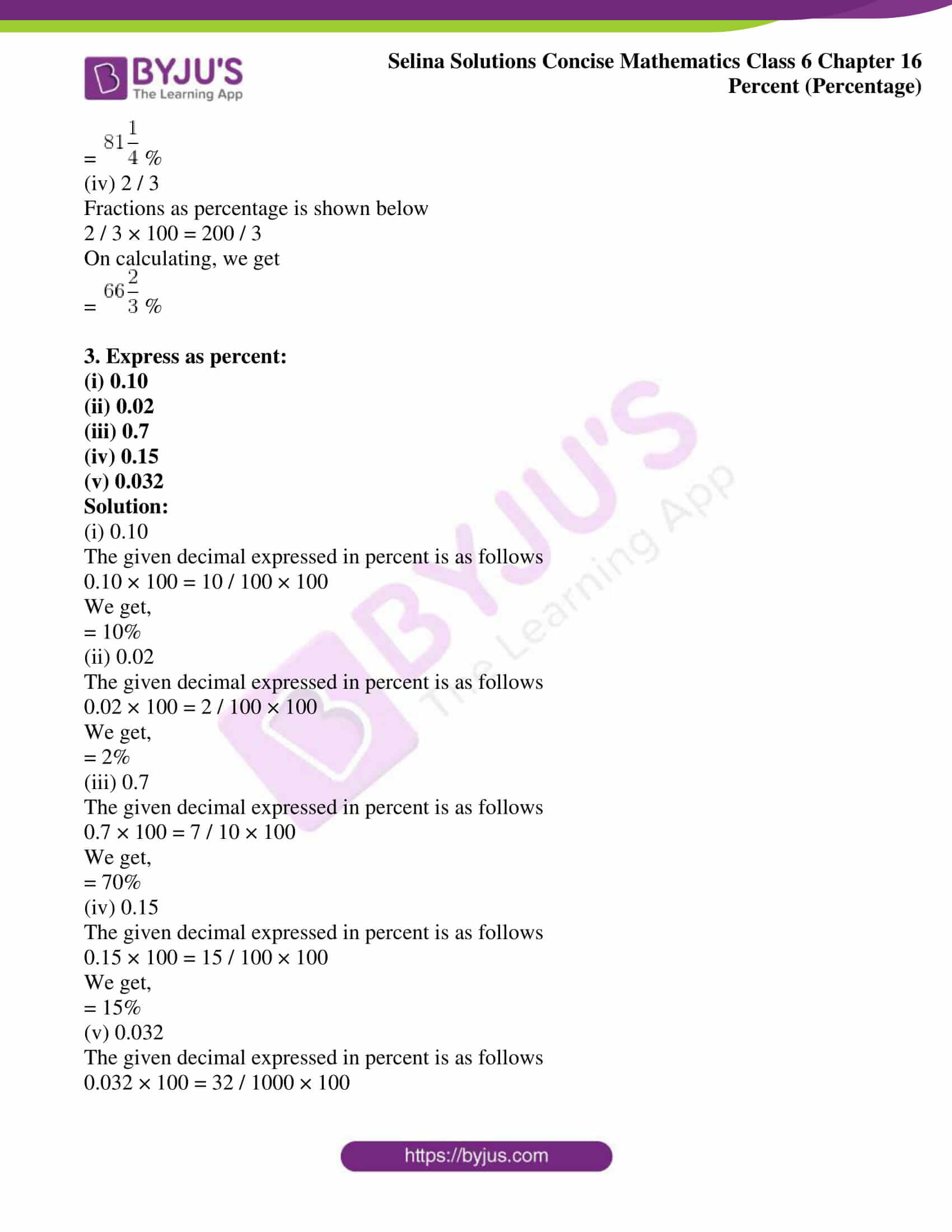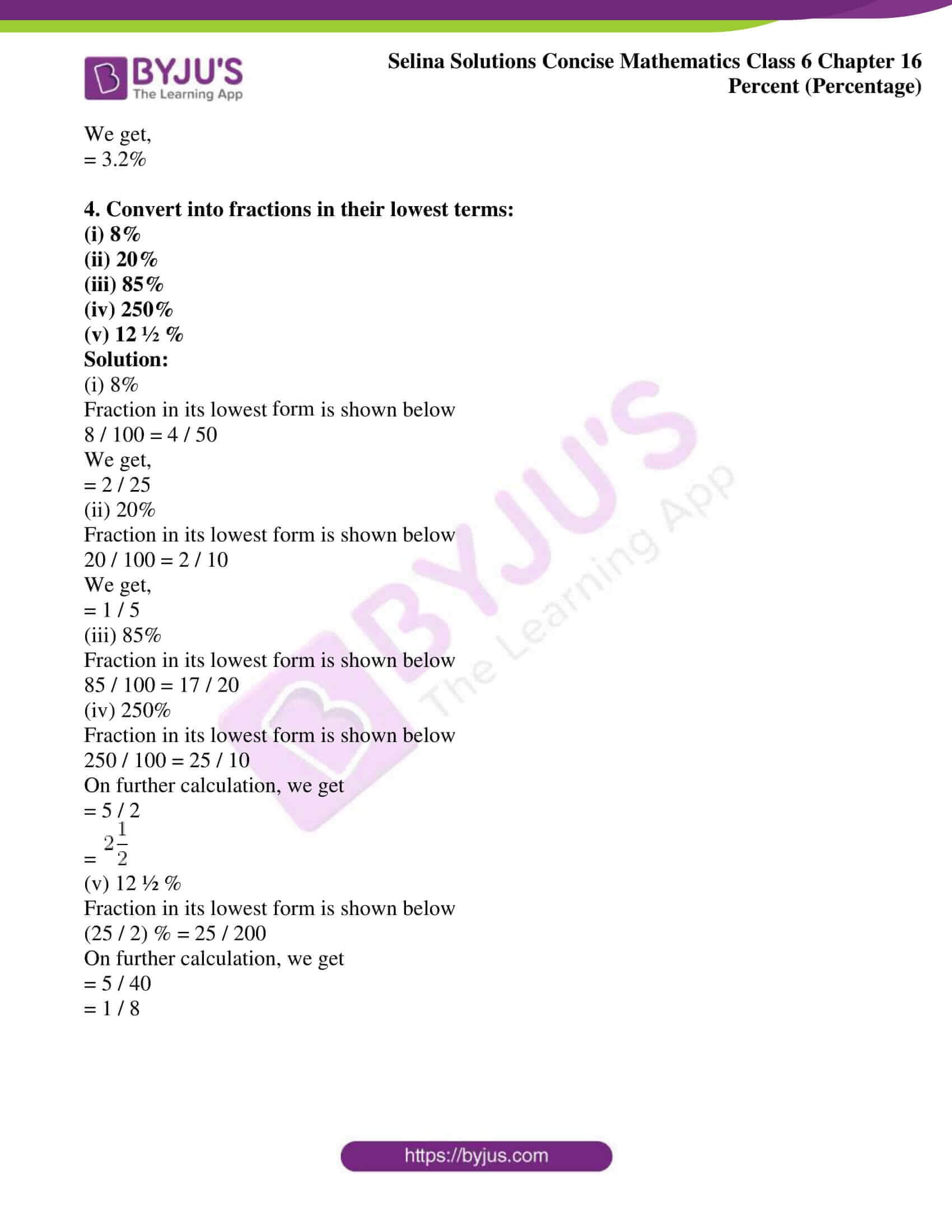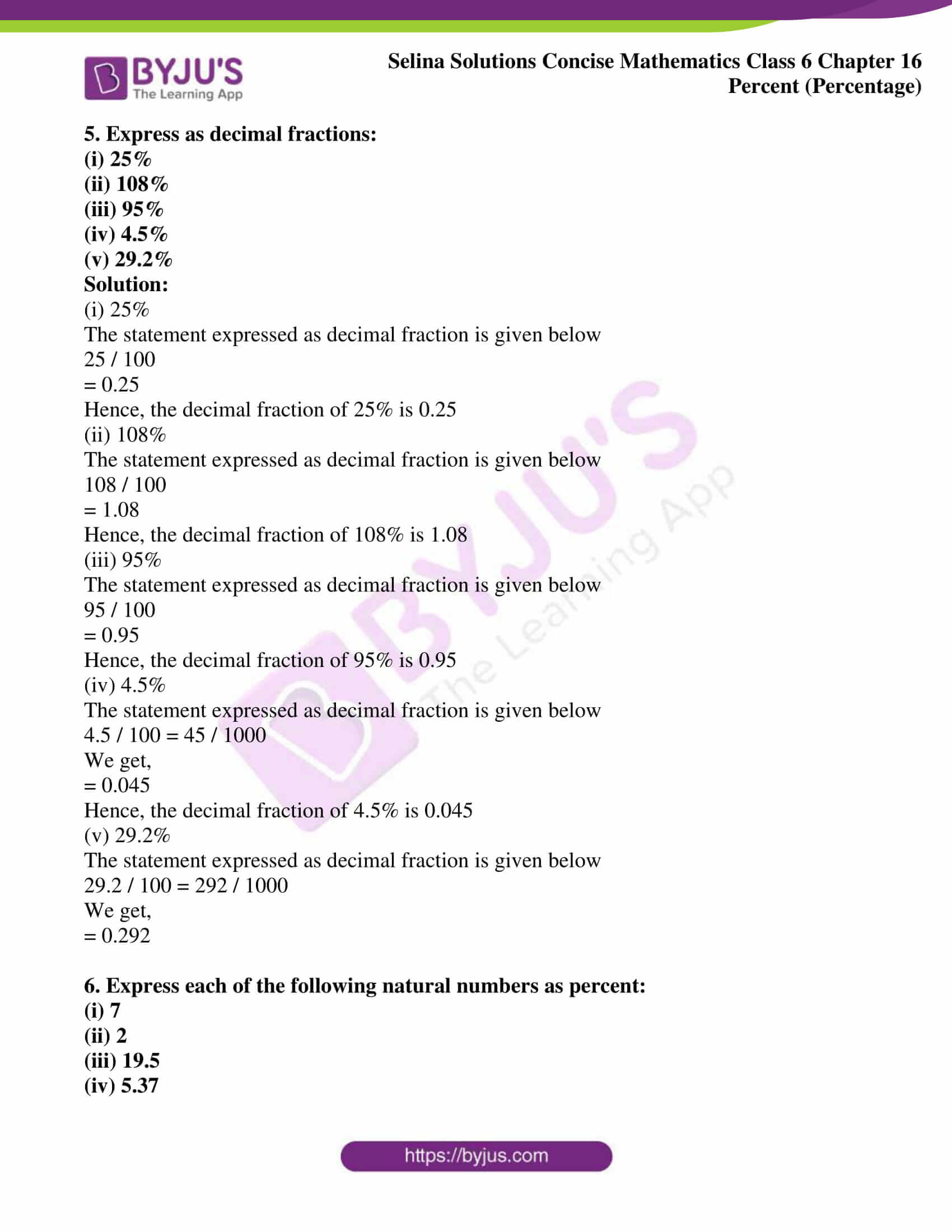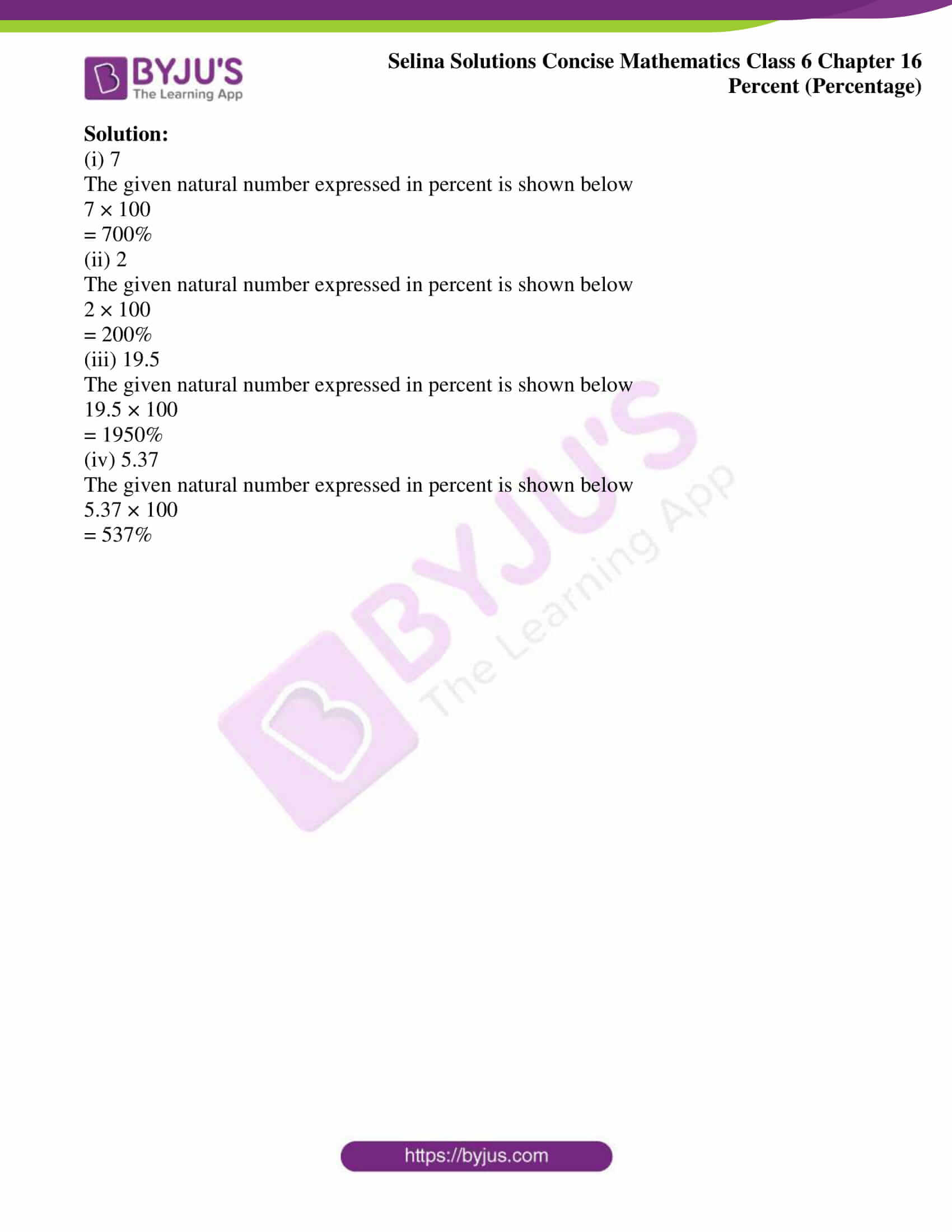### Access other exercises of Selina Solutions Concise Mathematics Class 6 Chapter 16: Percent (Percentage)

Exercise 16(B) Solutions

Exercise 16(C) Solutions

### Exercise 16(A)

1. Express each of the following statements in the percentage form:

(i) 13 out of 20

(ii) 21 eggs out of 30 are good

Solution:

(i) 13 out of 20

The statement in the percentage form is shown below

13 / 20 × 100 = 13 / 2 × 10

= 13 × 5

= 65%

(ii) The statement in the percentage form is shown below

21 / 30 × 100 = 21 / 3 × 10

= 7 × 10

= 70%

Hence, 70% of eggs are good

2. Express the following fractions as percent:

(i) 3 / 200

(ii) 5 / 6

(iii) 65 / 80

(iv) 2 / 3

Solution:

(i) 3 / 200

Fractions as percentage is shown below

3 / 200 × 100 = 3 / 2

We get,

= 1.5%

(ii) 5 / 6

Fractions as percentage is shown below

5 / 6 × 100 = 500 / 6

On calculating, we get

=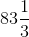%

(iii) 65 / 80

Fractions as percentage is shown below

65 / 80 × 100 = 65 / 8 × 10

= 325 / 4

On further calculation, we get

=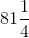%

(iv) 2 / 3

Fractions as percentage is shown below

2 / 3 × 100 = 200 / 3

On calculating, we get

=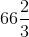%

3. Express as percent:

(i) 0.10

(ii) 0.02

(iii) 0.7

(iv) 0.15

(v) 0.032

Solution:

(i) 0.10

The given decimal expressed in percent is as follows

0.10 × 100 = 10 / 100 × 100

We get,

= 10%

(ii) 0.02

The given decimal expressed in percent is as follows

0.02 × 100 = 2 / 100 × 100

We get,

= 2%

(iii) 0.7

The given decimal expressed in percent is as follows

0.7 × 100 = 7 / 10 × 100

We get,

= 70%

(iv) 0.15

The given decimal expressed in percent is as follows

0.15 × 100 = 15 / 100 × 100

We get,

= 15%

(v) 0.032

The given decimal expressed in percent is as follows

0.032 × 100 = 32 / 1000 × 100

We get,

= 3.2%

4. Convert into fractions in their lowest terms:

(i) 8%

(ii) 20%

(iii) 85%

(iv) 250%

(v) 12 ½ %

Solution:

(i) 8%

Fraction in its lowest form is shown below

8 / 100 = 4 / 50

We get,

= 2 / 25

(ii) 20%

Fraction in its lowest form is shown below

20 / 100 = 2 / 10

We get,

= 1 / 5

(iii) 85%

Fraction in its lowest form is shown below

85 / 100 = 17 / 20

(iv) 250%

Fraction in its lowest form is shown below

250 / 100 = 25 / 10

On further calculation, we get

= 5 / 2

=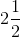(v) 12 ½ %

Fraction in its lowest form is shown below

(25 / 2) % = 25 / 200

On further calculation, we get

= 5 / 40

= 1 / 8

5. Express as decimal fractions:

(i) 25%

(ii) 108%

(iii) 95%

(iv) 4.5%

(v) 29.2%

Solution:

(i) 25%

The statement expressed as decimal fraction is given below

25 / 100

= 0.25

Hence, the decimal fraction of 25% is 0.25

(ii) 108%

The statement expressed as decimal fraction is given below

108 / 100

= 1.08

Hence, the decimal fraction of 108% is 1.08

(iii) 95%

The statement expressed as decimal fraction is given below

95 / 100

= 0.95

Hence, the decimal fraction of 95% is 0.95

(iv) 4.5%

The statement expressed as decimal fraction is given below

4.5 / 100 = 45 / 1000

We get,

= 0.045

Hence, the decimal fraction of 4.5% is 0.045

(v) 29.2%

The statement expressed as decimal fraction is given below

29.2 / 100 = 292 / 1000

We get,

= 0.292

6. Express each of the following natural numbers as percent:

(i) 7

(ii) 2

(iii) 19.5

(iv) 5.37

Solution:

(i) 7

The given natural number expressed in percent is shown below

7 × 100

= 700%

(ii) 2

The given natural number expressed in percent is shown below

2 × 100

= 200%

(iii) 19.5

The given natural number expressed in percent is shown below

19.5 × 100

= 1950%

(iv) 5.37

The given natural number expressed in percent is shown below

5.37 × 100

= 537%